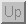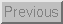Next: 4.7 Additional Signal Conditioning Up: 4 Signal Conditioning Previous: 4.5 Resistance to Voltage

## 4.6 Capacitance to Voltage

### 4.6.1 Motivation

We have seen that the electrical property of capacitance has been the main physical principle behind many of the sensors that we have discussed. The main reason why it is so useful is that it is a property which varies directly proportional to the distance between the metal plates. This has made it a useful tool in measuring small vibrations. Capacitance can also be used to measure much greater distances than we have seen so far. The Radio- Baton is an example of a system which uses capacitance to measure distances on the order of 1 meter.

Another useful property of capacitors is that they are sensitive to the material that resides between their metal plates. Specifically, it is the dielectric constant associated with the material that results in a change in capacitance. One example which makes use of this principle is that capacitors can be used as sensors which can detect the presence of an object between their plates. This principle can be used as a detector to determine when someone enters a space. In the case of the piezoelectric sensor, we used the fact that the voltage of a charged capacitor will vary inversely proportional to its capacitance. An op-amp circuit could then be used to amplify the voltage to a useable level. Since voltage and capacitance are inversely proportional, this is useful when small values of capacitance are to be measured. When one is dealing with larger values of capacitance the voltages resultant from a practical amount of charge are too small to be useful, and hence other methods must be used.

### 4.6.2 Circuits

Capacitance can be measured in the same two ways discussed previously for measuring resistance - a voltage divider or a bridge circuit. Instead of using resistors, capacitors are used. There is only one critical difference: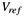must be a sinusoidal signal since the capacitor blocks DC. Since the impedance of a capacitor is inversely proportional to frequency, the HCI designer should choose a frequency forthat creates a voltage across the capacitor that is appropriate for amplification (i.e, too small a frequency will cause too high of an impedance of the sensor which will cause too much noise from the sensor; too high of a frequency will cause too low of an impedance of the sensor which will cause other circuit noise to swamp out the voltage across the sensor).

### 4.6.3 Examples

The ADXL50 accelerometer provides as its output a voltage level which varies in proportion to the amount of acceleration experienced along its sensitive axis. It is calibrated such that under no acceleration the output will be 1.8 volts. Furthermore, an acceleration of 50g (where 1g is the acceleration due to gravity) will cause a voltage swing of 1volt (ie. an acceleration of 50g would result in an output of either 2.8volts, or .8 volts depending upon the direction). In many cases this voltage swing would not be appropriate for a specific application, hence one must use a circuit which converts the output voltage into a more useful voltage range. For example, one might desire a more sensitive device which varied 1volt for every g of acceleration. Figure 39 depicts a circuit which accomplishes this task.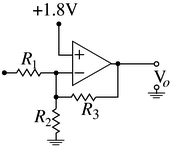Figure 39: Schematic of an op-amp circuit which converts the output voltage of the ADXL-50 to a range which is suitable for analog-digital conversion.

The output voltage of this circuit is given by:When no acceleration is applied to the device, the output voltage will be 1.8volts which makes the second term will be zero, and the output will be, the new zero-g output. The output voltage can be written as: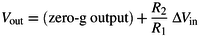where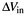is the deviation of the output voltage from 1.8 volts. A 50g acceleration will result inof 1volt, which will be scaled by. In this way, we see thatdetermines the sensitivity of the output, while the new zero-g output is determined by.Next: 4.7 Additional Signal Conditioning Up: 4 Signal Conditioning Previous: 4.5 Resistance to Voltage

Tim Stilson
Thu Oct 17 16:32:33 PDT 1996